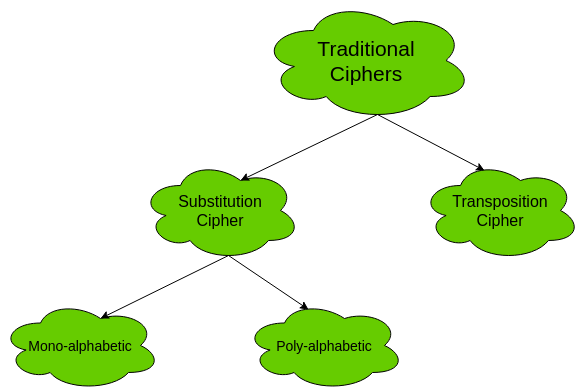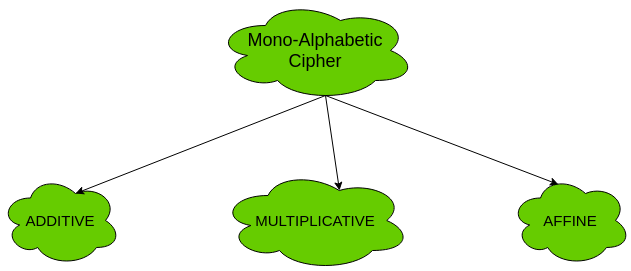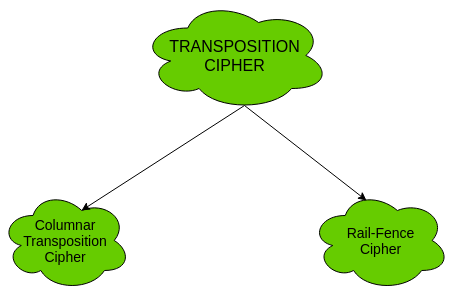Related Articles
• Explore Our Geeks Community
• Cryptography Tutorial

The two types of traditional symmetric ciphers are Substitution Cipher and Transposition Cipher. The following flowchart categories the traditional ciphers:1. Substitution Cipher:
Substitution Ciphers are further divided into Mono-alphabetic Cipher and Poly-alphabetic Cipher.

First, let’s study about mono-alphabetic cipher.

1. Mono-alphabetic Cipher –
In mono-alphabetic ciphers, each symbol in plain-text (eg; ‘o’ in ‘follow’) is mapped to one cipher-text symbol. No matter how many times a symbol occurs in the plain-text, it will correspond to the same cipher-text symbol. For example, if the plain-text is ‘follow’ and the mapping is :

• f -> g
• o -> p
• l -> m
• w -> x

The cipher-text is ‘gpmmpx’.

Types of mono-alphabetic ciphers are:(a). Additive Cipher (Shift Cipher / Caesar Cipher) –
The simplest mono-alphabetic cipher is additive cipher. It is also referred to as ‘Shift Cipher’ or ‘Caesar Cipher’. As the name suggests, ‘addition modulus 2’ operation is performed on the plain-text to obtain a cipher-text.

C = (M + k) mod n
M = (C – k) mod n

where,
C -> cipher-text
M -> message/plain-text
k -> key

The key space is 26. Thus, it is not very secure. It can be broken by brute-force attack.

(b). Multiplicative Cipher –
The multiplicative cipher is similar to additive cipher except the fact that the key bit is multiplied to the plain-text symbol during encryption. Likewise, the cipher-text is multiplied by the multiplicative inverse of key for decryption to obtain back the plain-text.

C = (M * k) mod n
M = (C * k-1) mod n

where,
k-1 -> multiplicative inverse of k (key)

The key space of multiplicative cipher is 12. Thus, it is also not very secure.

(c). Affine Cipher –
The affine cipher is a combination of additive cipher and multiplicative cipher. The key space is 26 * 12 (key space of additive * key space of multiplicative) i.e. 312. It is relatively secure than the above two as the key space is larger.
Here two keys k1 and k2 are used.

C = [(M * k1) + k2] mod n
M = [(C – k2) * k1-1 ] mod n

Now, let’s study about poly-alphabetic cipher.

2. Poly-alphabetic Cipher –
In poly-alphabetic ciphers, every symbol in plain-text is mapped to a different cipher-text symbol regardless of its occurrence. Every different occurrence of a symbol has different mapping to a cipher-text. For example, in the plain-text ‘follow’, the mapping is :

f -> q
o -> w
l -> e
l -> r
o -> t
w -> y

Thus, the cipher text is ‘qwerty’.

Types of poly-alphabetic ciphers are:2. Transposition Cipher:
The transposition cipher does not deal with substitution of one symbol with another. It focuses on changing the position of the symbol in the plain-text. A symbol in the first position in plain-text may occur in fifth position in cipher-text.

Two of the transposition ciphers are:1. Columnar Transposition Cipher –
For information and implementation, see Columnar Transposition Cipher
2. Rail-Fence Cipher –
For information and implementation, see Rail-Fence Cipher
Unlock the Power of Placement Preparation!
Feeling lost in OS, DBMS, CN, SQL, and DSA chaos? Our Complete Interview Preparation Course is the ultimate guide to conquer placements. Trusted by over 100,000+ geeks, this course is your roadmap to interview triumph.